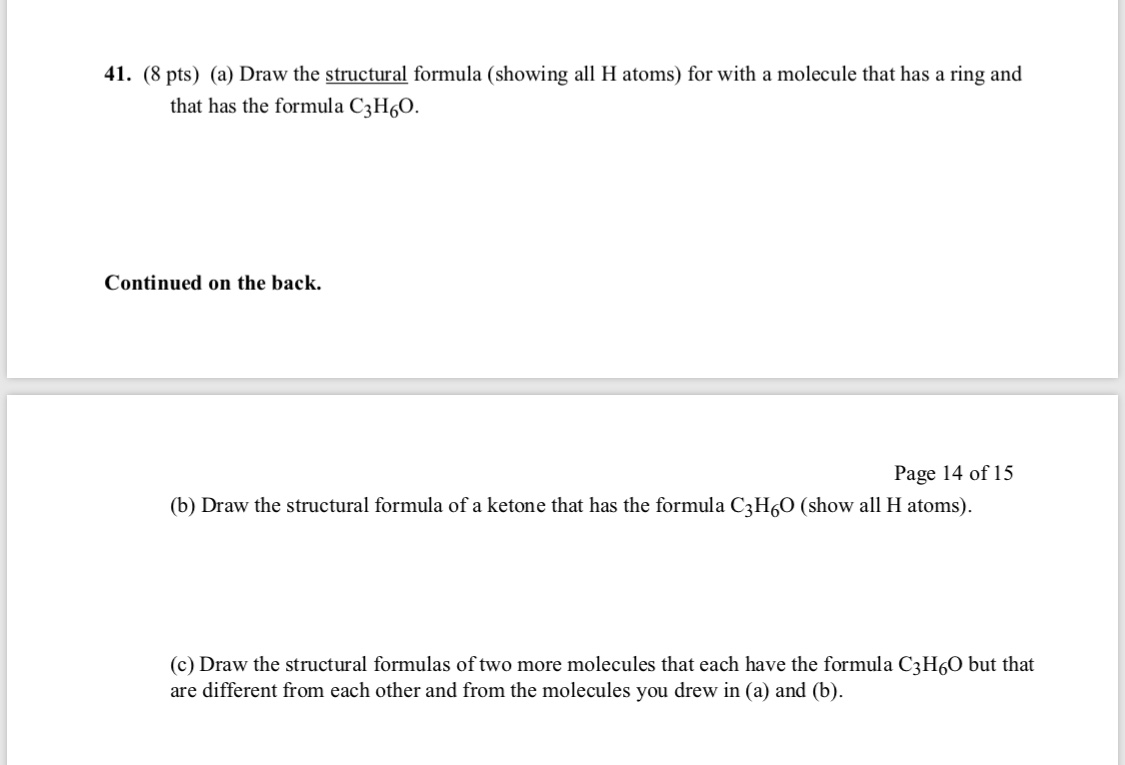# (a) Draw the structural formula (showing all H atoms) for with a molecule that has a ring and that has the formula C3H6O. (b) Draw the structural formula of a ketone that has the formula C3H6O (showing all H atoms). (c) Draw the structural formulas of two more molecules that each have the formula C3H6O but that are different from each other and from the molecules you drew in (a) and (b).